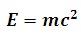# Einstein Mass Energy Caculator

This Calctown Calculator calculates the energy equivalence of a given mass.

kg

#### Result

Nwhere E = Eqiuvalent energy m = given mass c = speed of light in vacuum